# gghighlight 0.3.2

gghighlight
ggplot2
R package
Author

Hiroaki Yutani

Published

June 7, 2021

gghighlight 0.3.2 is on CRAN now!

This release is mainly for fixing the potential test failures with upcoming version of ggplot2, but this version contains two new features.

## `n()`

Since gghighlight uses dplyr inside, you can now use dplyr’s expression, `n()`. This is useful to highlight based on the size of the group.

Suppose we have this data:

``library(gghighlight)``
``Loading required package: ggplot2``
``````library(dplyr, warn.conflicts = FALSE)

set.seed(1098)
centers <- tibble(
id = sample(letters, 11),
x = c(-1, -2, -3,   0,  1,  4,  2,  5,  7,  1, -1),
y = c( 4, -3, -7, -10, -8, -3,  9,  5, -1,  1, -1),
n = c(50, 50, 100, 50, 50, 120, 40, 10, 20, 5, 8)
)

d <- centers %>%
rowwise() %>%
summarise(id = id, x = x + rnorm(n, sd = 3), y = y + rnorm(n, sd = 3))

p <- ggplot(d, aes(x, y, colour = id)) + geom_point()
p``````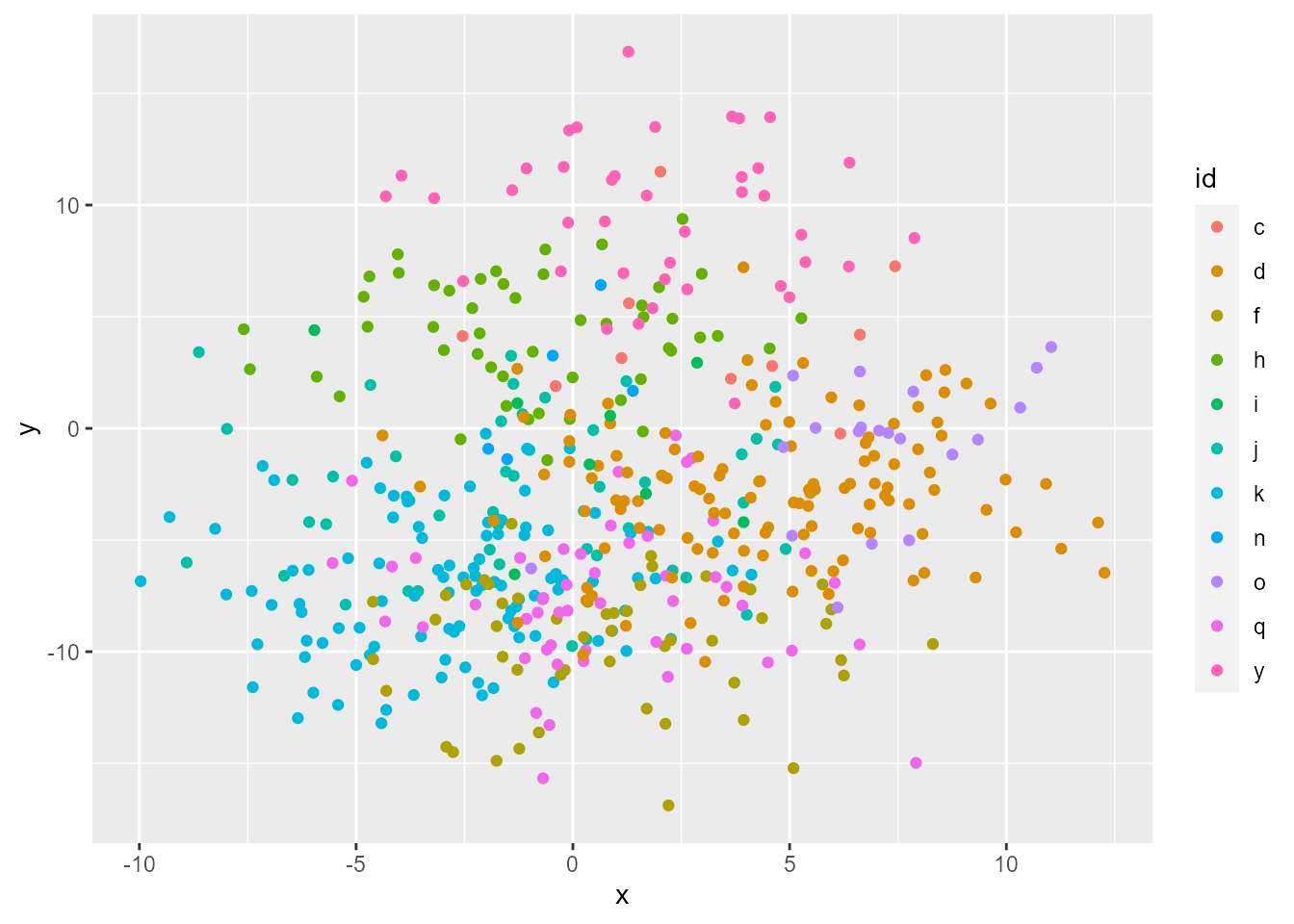By using `n()`, we can focus on the large groups.

``````p +
gghighlight(n() >= 100, use_direct_label = FALSE)``````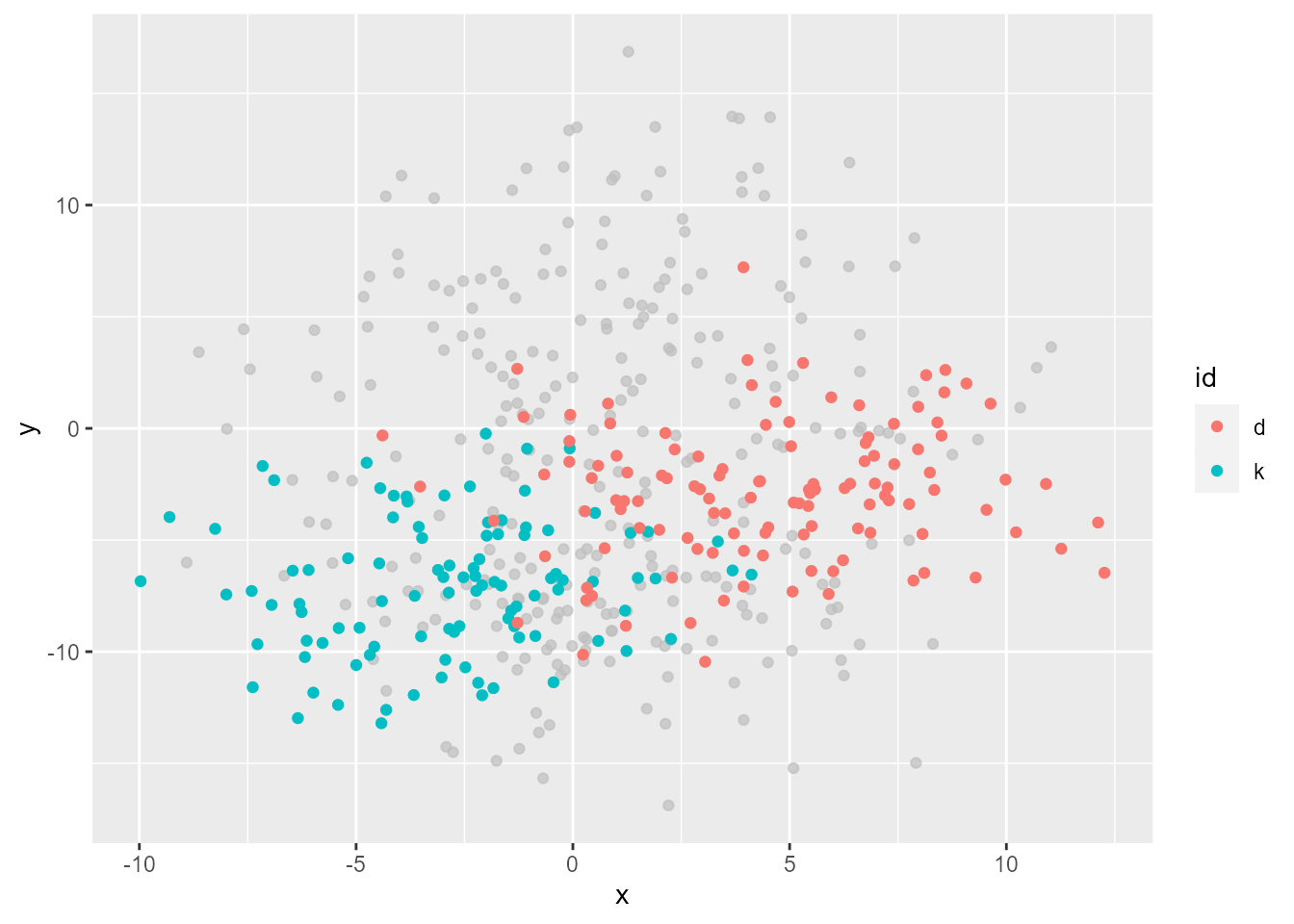Or small groups.

``````p +
gghighlight(n() < 10, use_direct_label = FALSE)``````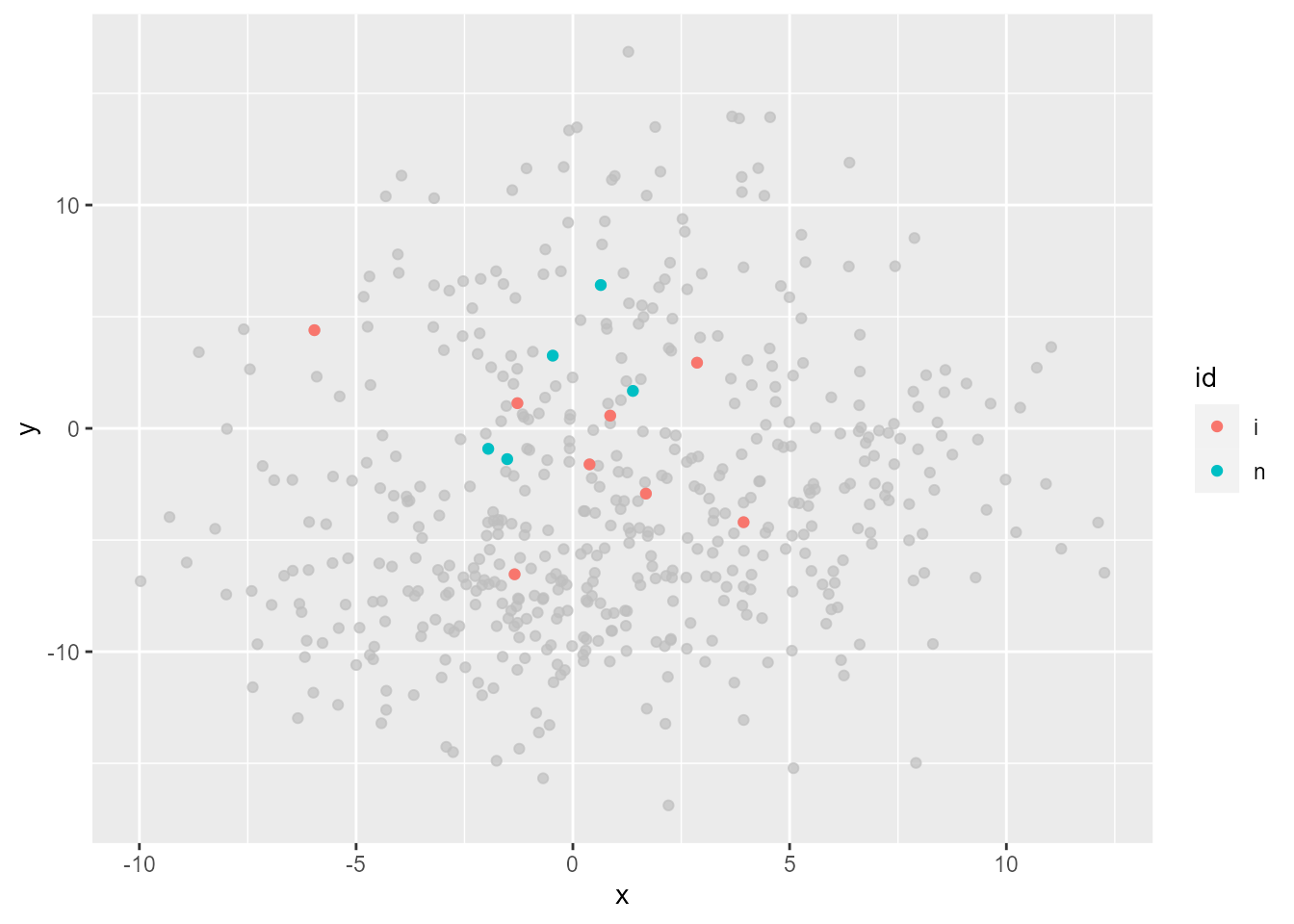You can also use `n()` as a non-logical predicate, whose values are used for sorting data and the top `max_highlight` of rows/groups are highlighted.

``````# Same result as above
p +
gghighlight(-n(), max_highlight = 2, use_direct_label = FALSE)``````

## To unhighlight or not to unhighlight…

By default, unhighlighted data are grayed out. `unhighlighted_params` is the option to override this. Now, you can even choose not to unhighlight at all by specifying explicit `NULL` to `colour` or `fill`!

``````p +
gghighlight(n() < 10, use_direct_label = FALSE,
unhighlighted_params = list(colour = NULL))``````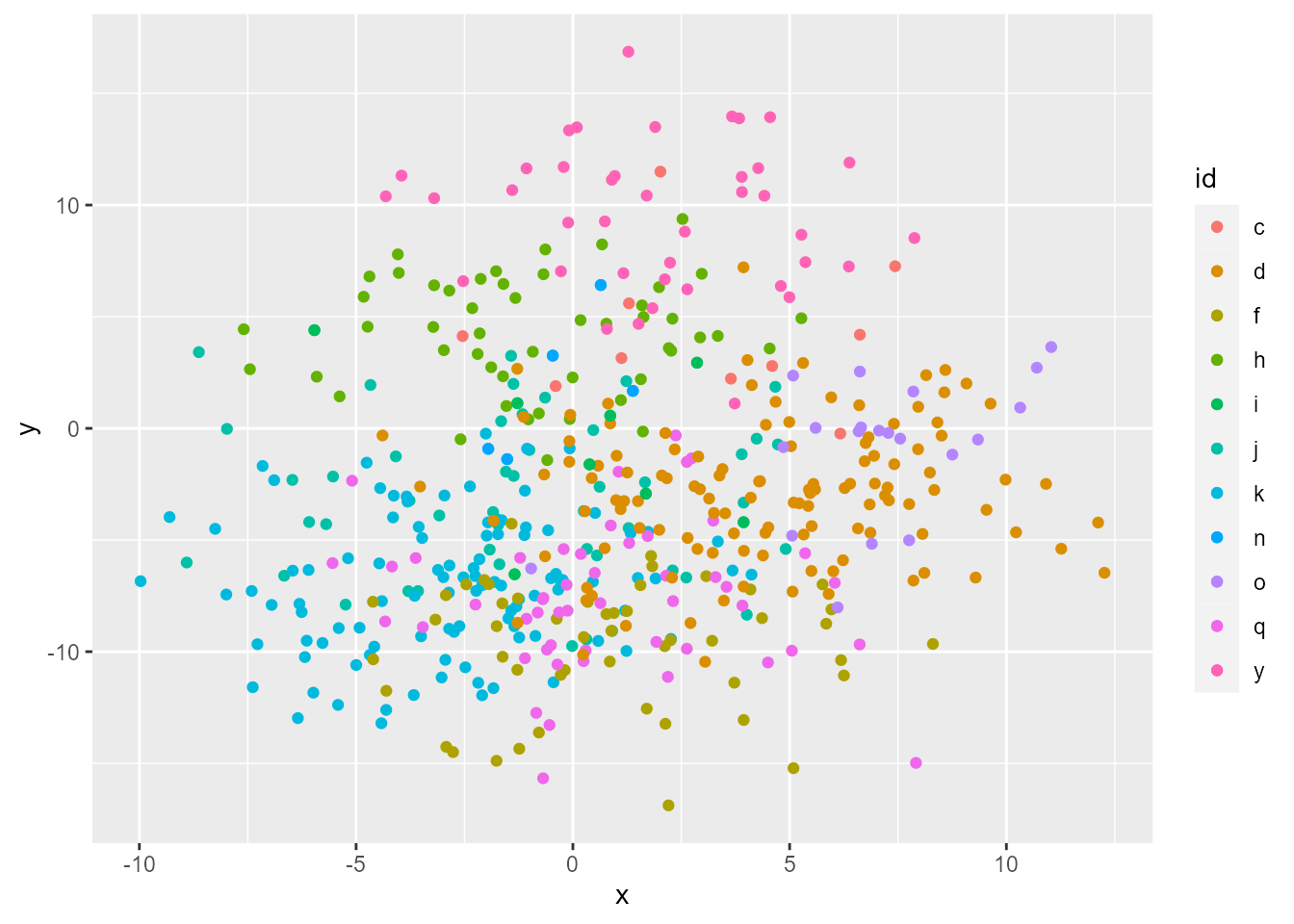Hmm…, but this is the very same plot as the original one. How can this be useful? Well, remember we still can tweak other parameters like `alpha`.

``````p +
gghighlight(n() < 10, use_direct_label = FALSE,
unhighlighted_params = list(colour = NULL, alpha = 0.2))``````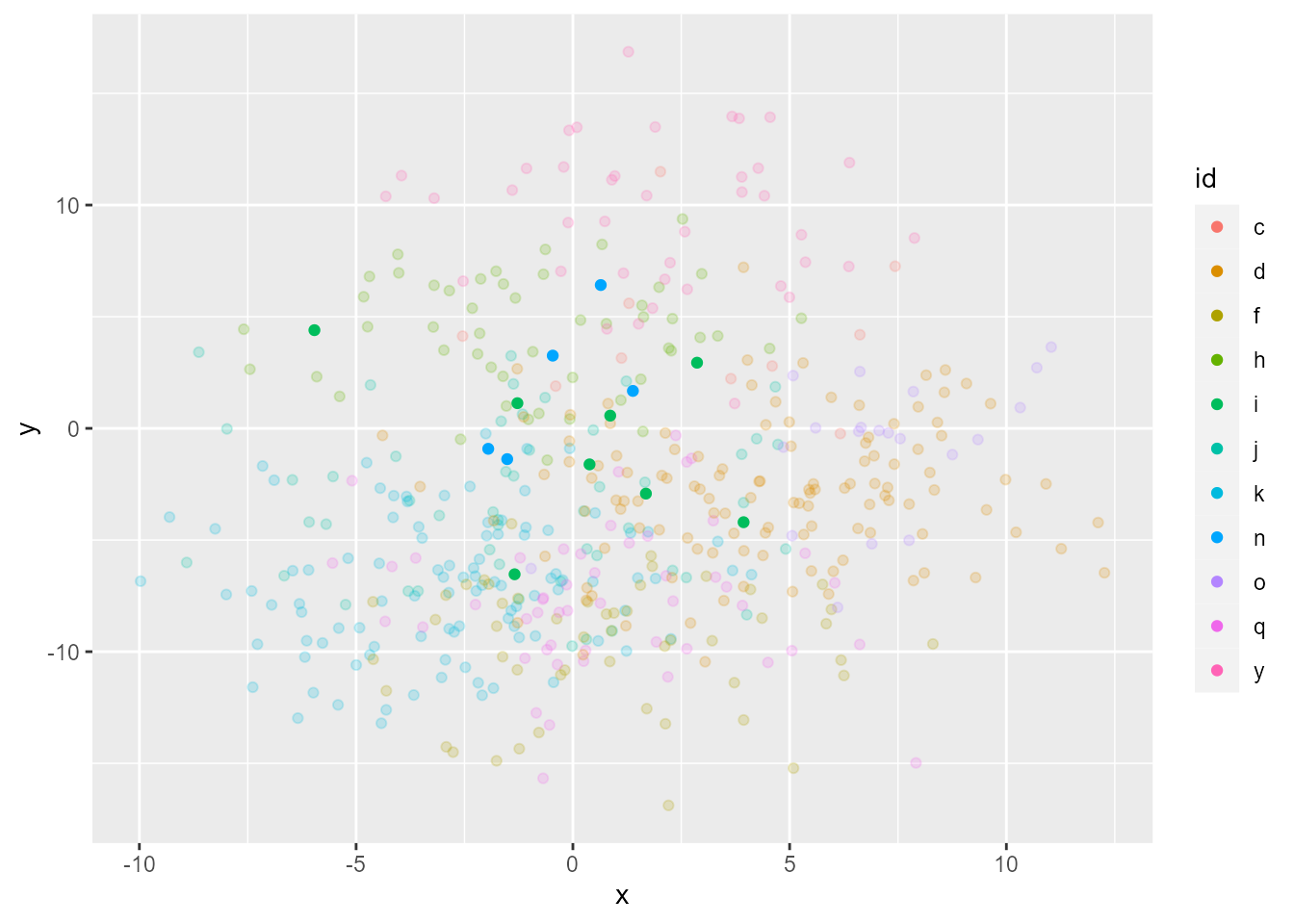This plot doesn’t look very nice in that the colors are a bit difficult to distinguish. This is mainly because I didn’t come up with some nice data, but it’s generally a tough job to tweak colors by `alpha` properly, so I don’t recommend this much. But, hope you can find some good use case for this!